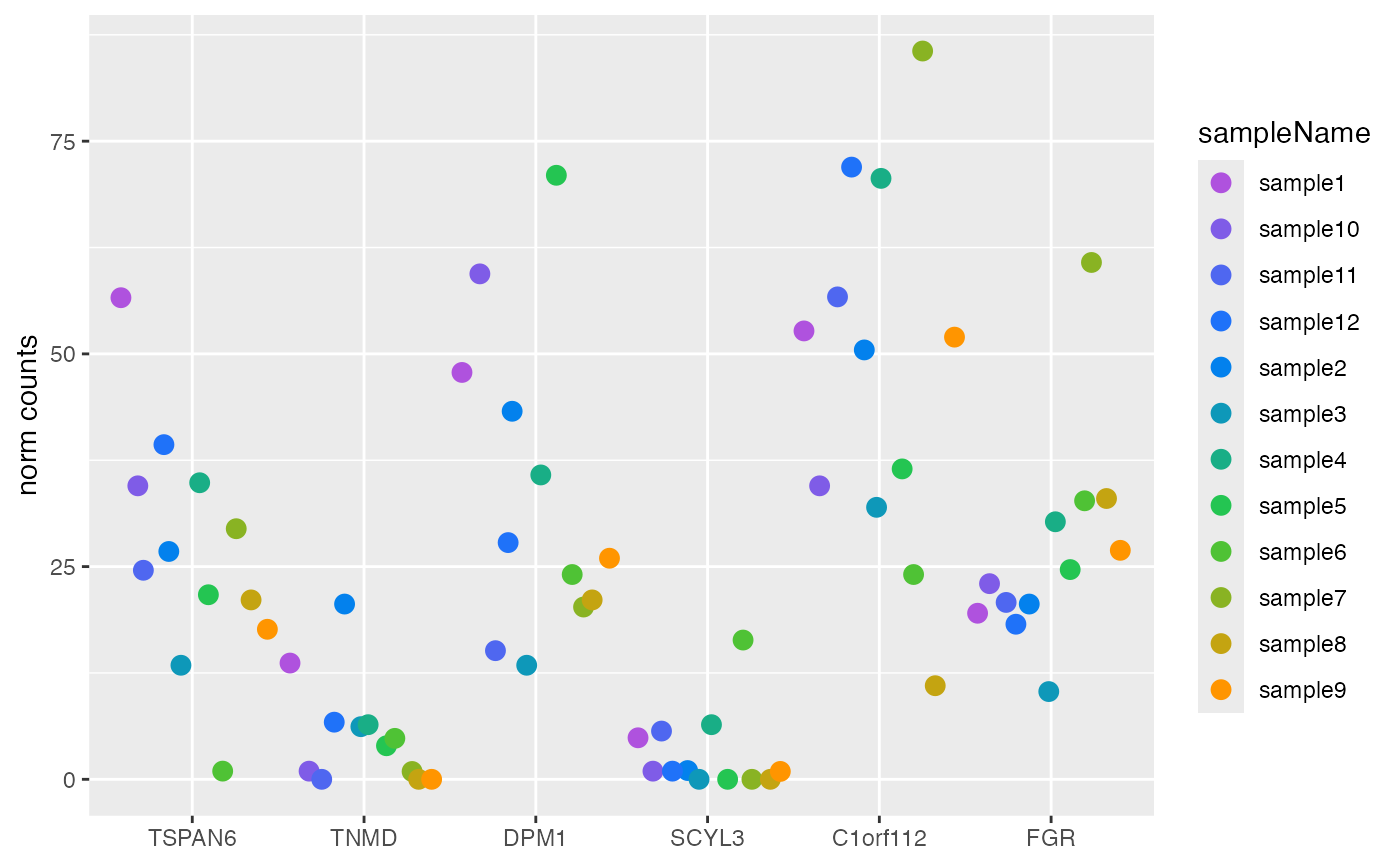Plot counts

plotCounts(object, ...)

# S4 method for DESeqAnalysis
plotCounts(object, ..., samples = NULL, transform = FALSE)

# S4 method for DESeqDataSet
plotCounts(object, ...)

# S4 method for DESeqTransform
plotCounts(object, ...)

Arguments

object Object. Additional arguments. character. Sample identifiers. logical(1). Plot log2 variance-stabilized transform counts, defined internally in DESeqTransform object (see transform slot). If FALSE, plot the size factor adjusted counts from DESeqDataSet.

Value

• style = "facet": ggplot grouped by sampleName, with ggplot2::facet_wrap() applied to panel the samples.

• style = "wide": ggplot in wide format, with genes on the x-axis.

Functions

• plotCounts,DESeqAnalysis-method: Plot either DESeqDataSet normalized counts or DESeqTransform log2 variance-stabilized counts.

• plotCounts,DESeqDataSet-method: Plot size factor (i.e. library size) adjusted normalized counts. Arguments pass through to SummarizedExperiment method defined in AcidPlots package.

• plotCounts,DESeqTransform-method: Plot log2 variance-stabilized transformed counts.

Note

Updated 2021-03-15.